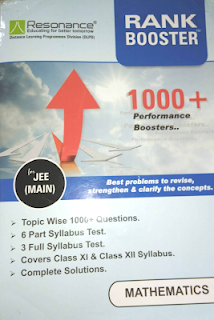Home COACHING MATERIALS RESONANCE RANK BOOSTER JEE MAIN PDF

# RESONANCE RANK BOOSTER JEE MAIN PDF

Hi guys, welcome back to iitianscareer.com

## RESONANCE RANK BOOSTER JEE MAIN PDF

#### ☆ THE CONTENT OF RESONANCE RANK BOOSTER “PHYSICS” MODULE:-

1. Unit and dimension
2. Error in measurement
3. Rectilinear motion
4. Projectile motion
5. Relative motion
6. Newton’s law of motion
7. Friction
8. Work, power & energy
9. Circular motion
10. Centre of mass
11. Rigid body dynamics
12. Simple harmonic motion
13. Wave on a string
14. Sound waves
15. Heat and thermodynamics
16. Calorimetry and thermal expansion
17. Fluid mechanics, elasticity, viscosity and surface tension
18. Geometrical optics
19. Electrostatics
20. Gravitation
21. Current electricity
22. Heat transfer
23. Capacitance
24. Electromagnetic field
25. Electromagnetic induction
26. Alternating current
27. Wave optics
28. Modern physics
29. Nuclear physics
30. Solid and semiconductor devices
31. Electromagnetic waves and communication

# “ALLEN PHYSICS CHAPTERWISE SHEETS”

#### ☆ THE CONTENT OF RESONANCE RANK BOOSTER “CHEMISTRY” MODULE:-

1. Stoichiometry
2. Atomic structure
3. Gaseous state
4. Chemical equilibrium
5. Ionic equilibrium
6. Thermodynamics and thermochemistry
8. Electrochemistry
9. Solid state
10. Solution and colligative properties
11. Surface chemistry
12. Periodic table & periodicity
13. Chemical bonding
14. Coordination compounds
15. Metallurgy
16. S block elements
17. P block elements
18. D and F block elements
19. Qualitative analysis
20. Hydrogen, its compounds and environmental chemistry
21. Basic concepts of organic chemistry, IUPAC nomenclature, isomerism and seperation techniques
22. Fundamental concepts in organic reaction mechanism
23. Hydrocarbon
24. Alkyl hallide, alcohol, phenol, ether
25. Aldehydes, ketones, carboxylic acid and their derivatives
26. Nitrogen containing compounds
27. Biomolecules and polymers
28. Chemistry in everyday life

# “40 YEARS CHEMISTRY OF IIT JEE BY DISHA PUBLICATIONS“

###### ☆ CONTENT OF RESONANCE RANK BOOSTER “MATHEMATICS” MODULE:-

1. Straight line
2. Circle
3. Parabola
4. Ellipse
5. Hyperbola
6. Set and relation
7. Function
8. Limit of function
9. Continuity and differentiablity
10. Method of differentiation
11. Application of derivatives
12. Indefinite integral
13. Definite integral
14. Area under the curve
15. Differential equations
17. Sequence and series
18. Binomial theorem and mathematical induction
19. Permutation & combination
20. Probability
21. Matrices and determinants
22. Complex number
23. Vectors
24. 3Dimensional geometry
25. Solution of triangles, Height & Distance
26. Trigonometric identities and equations
27. Inverse trigonometric functions
28. Statistics
29. Mathematical reasoning